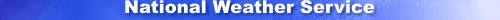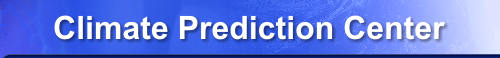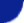efficiently. Climate Prediction Center - wgrib2: -set_bin_precSite Map News Organization SearchHOME > Monitoring_and_Data > Oceanic and Atmospheric Data > Reanalysis: Atmospheric Data > wgrib2 -set_bin_prec

Introduction

The values at the grid points points are stared in a general format,

Y = (R + i*2**B)*(10**D)

R = reference value (32-bit IEEE floating point number)
i = integer, 0..2**N-1
N = binary bit precision
B = binary scaling, -127..127
D = decimal scaling, -127..127
There are 3 sytems for storing the number which I have called

ECMWF convention: D = 0, N = parameter

Y = R + i*2**B
R = reference value
i = integer, 0..2**N-1
N = binary bit precision, a parameter
B = binary scaling, determined by grib routines

NCEP convention: B = parameter, D = parameter

R = reference value
i = integer, 0..2**N-1
N = binary bit precision, determined by grib routines
B = binary scaling, a parameter
D = decimal scaling, a parameter

Note, global model uses a variant: B = 0, D = parameter

Both the ECMWF and NCEP conventions have their advantages and disadvantages. The ECMWF method is easier to use, you just set the binary precision to N bits (12? 16?) for all variables and you are done. With the NCEP convention, you have to set the scaling for each variable separately. For some variables such as specific humidity, the scaling should be pressure dependent. On the other hand, if you are trying to get the smallest files, the NCEP convention is better. For example, you want to get the RH to the nearest integer. With the NCEP method, you simply set D = B = 0. For general use, I suggest that you use the ECMWF convention because people time is usually more valuable than disk space. Ok, I value my time more than a few GB. On the othe hand, I've been involved with more than my share of projects were disk space has been the critical issue.

By the way, the wgrib2 default for encoding grib is 12-bits using the ECMWF convention.

Usage

The -set_bin_prec option is used to set wgrib2 to encode data using the ECMWF convention.

-set_bin_prec N
N = number of bits to encode grid point data
N should be ≤ 25 for the current version of wgrib2

if N is > 12, you need to increase the maximum bits of allowed
precision by -set_grib_max_bits
 NOAA/ National Weather Service National Centers for Environmental Prediction Climate Prediction Center 5830 University Research Court College Park, Maryland 20740 Climate Prediction Center Web Team Page last modified: Dec 21, 2015, Marcy 11, 2019 Disclaimer Privacy Policy